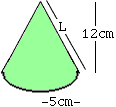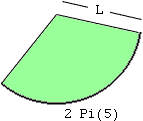Name: frothy60
Level: Middle

Question:
I don't understand how to find the surface area of a cone. The height is 12cm and the radius is 5cm. Thanks

Hi,For the slanting surface: imagine you cut down the cone from the point (apex) straight to the base and open it up onto a plane. You will have piece of a larger circle (radius the slant height). You will get the slant-height (the length of the cut) as the hypotenuse of a right triangle with sides: radius (5cm) and height (12cm). With Pythagoris' Theorem, you calculate the slant height L.The next question is: what fraction of this large circle to you have. You will then take the area of the entire large circle and multiply by this fraction to get the area of the surface of the cone.    How do we measure 'fractions' of a circle? One way is the angle (in degrees) divided by the angle of the whole (360). A second way, which will work better here, is the length of the arc of the piece you want over the length of the whole circumference of the circle. The whole circumference is 2 Pi L. The length of the arc you want is the same as the length of the circle around the base of the cone which you cut: 2 Pi (5).